# Selina solutions for Concise Maths Class 10 ICSE chapter 13 - Section and Mid-Point Formula [Latest edition]

#### Chapters## Chapter 13: Section and Mid-Point Formula

Exercise 13 (A)Exercise 13 (B)Exercise 13 (C)
Exercise 13 (A) [Pages 177 - 178]

### Selina solutions for Concise Maths Class 10 ICSE Chapter 13 Section and Mid-Point Formula Exercise 13 (A) [Pages 177 - 178]

Exercise 13 (A) | Q 1.1 | Page 177

Calculate the co-ordinates of the point P which divides the line segment joining: A (1, 3) and B (5, 9) in the ratio 1 : 2

Exercise 13 (A) | Q 1.2 | Page 177

Calculate the co-ordinates of the point P which divides the line segment joining:  A (-4, 6) and B(3, -5) in the ratio 3 : 2

Exercise 13 (A) | Q 2 | Page 177

In what ratio is the line joining (2, -3) and (5, 6) divided by the x-axis.

Exercise 13 (A) | Q 3 | Page 177

In what ratio is the line joining (2, -4) and (-3, 6) divided by the y – axis.

Exercise 13 (A) | Q 4 | Page 177

In what ratio does the point (1, a) divide the join of (-1, 4) and (4,-1)? Also, find the value of a.

Exercise 13 (A) | Q 5 | Page 177

In what ratio does the point (a, 6) divide the join of (-4, 3) and (2, 8)? Also, find the value of a.

Exercise 13 (A) | Q 6 | Page 177

In what ratio is the join of (4, 3) and (2, -6) divided by the x-axis. Also, find the co-ordinates of the point of intersection.

Exercise 13 (A) | Q 7 | Page 177

Find the ratio in which the join of (-4, 7) and (3, 0) is divided by the y-axis. Also, find the coordinates of the point of intersection.

Exercise 13 (A) | Q 8 | Page 177

Points A, B, C and D divide the line segment joining the point (5, -10) and the origin in five equal parts. Find the co-ordinates of B and D.

Exercise 13 (A) | Q 9 | Page 177

The line joining the points A (-3, -10) and B (-2, 6) is divided by the point P such that (PB)/(AB)=1/5 Find the co-ordinates of P.

Exercise 13 (A) | Q 10 | Page 177

P is a point on the line joining A(4, 3) and B(-2, 6) such that 5AP = 2BP. Find the co-ordinates of P.

Exercise 13 (A) | Q 11 | Page 177

Calculate the ratio in which the line joining the points (-3, -1) and (5, 7) is divided by the line x = 2. Also, find the co-ordinates of the point of intersection.

Exercise 13 (A) | Q 12 | Page 177

Calculate the ratio in which the line joining A(6, 5) and B(4, -3) is divided by the line y = 2.

Exercise 13 (A) | Q 13 | Page 177

The point P (5, -4) divides the line segment AB, as shown in the figure, in the ratio 2 : 5. Find the co-ordinates of points A and B.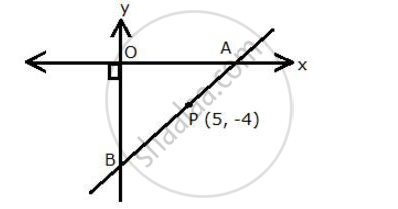Exercise 13 (A) | Q 14 | Page 177

Find the co-ordinates of the points of tri-section of the line joining the points (-3, 0) and (6, 6)

Exercise 13 (A) | Q 15 | Page 177

Show that the line segment joining the points (-5, 8) and (10, -4) is trisected by the co-ordinate axes.

Exercise 13 (A) | Q 16 | Page 177

Show that A (3, -2) is a point of trisection of the line segment joining the points (2, 1) and (5, -8).
Also, find the co-ordinates of the other point of trisection.

Exercise 13 (A) | Q 17 | Page 177

If A = (-4, 3) and B = (8, -6)
(i) Find the length of AB
(ii) In what ratio is the line joining A and B, divided by the x-axis?

Exercise 13 (A) | Q 18 | Page 177

The line segment joining the points M(5, 7) and N(-3, 2) is intersected by the y-axis at point L. Write down the abscissa of L. Hence, find the ratio in which L divides MN. Also, find the co-ordinates of L.

Exercise 13 (A) | Q 19 | Page 177

A (2, 5), B (-1, 2) and C (5, 8) are the co-ordinates of the vertices of the triangle ABC. Points P
and Q lie on AB and AC respectively,
Such that: AP : PB = AQ : QC = 1 : 2
(i) Calculate the co-ordinates of P and Q.
(ii) Show that PQ =1/3BC

Exercise 13 (A) | Q 20 | Page 177

A (-3, 4), B (3, -1) and C (-2, 4) are the vertices of a triangle ABC. Find the length of line segment AP, where point P lies inside BC, such that BP : PC = 2 : 3

Exercise 13 (A) | Q 21 | Page 177

The line segment joining A (2, 3) and B (6, -5) is intercepted by x-axis at the point K. Write down the ordinate of the point K. Hence, find the ratio in which K divides AB. Also find the coordinates of the point K.

Exercise 13 (A) | Q 22 | Page 177

The line segment joining A (4, 7) and B (-6, -2) is intercepted by the y – axis at the point K. write down the abscissa of the point K. hence, find the ratio in which K divides AB. Also, find the co-ordinates of the point K.

Exercise 13 (A) | Q 23 | Page 177

The line joining P(-4, 5) and Q(3, 2) intersects the y-axis at point R. PM and QN are perpendicular from P and Q on the x-axis Find:

(i) the ratio PR : RQ

(ii) the coordinates of R.

(iii) the area of the quadrilateral PMNQ.

Exercise 13 (A) | Q 24 | Page 177

In the given figure line APB meets the x-axis at point A and y-axis at point B. P is the point (-4,2) and AP : PB = 1 : 2. Find the co-ordinates of A and B.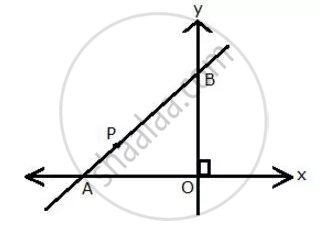Exercise 13 (A) | Q 25 | Page 177

Given a line segment AB joining the points A (-4, 6) and B (8, -3). Find:
(i) the ratio in which AB is divided by the y-axis
(ii) find the coordinates of the point of intersection
(iii) the length of AB.

Exercise 13 (A) | Q 26 | Page 178

If P(-b, 9a - 2) divides the line segment joining the points A(-3, 3a + 1) and B(5, 8a) in the ratio 3: 1, find the values of a and b.

Exercise 13 (B) [Page 182]

### Selina solutions for Concise Maths Class 10 ICSE Chapter 13 Section and Mid-Point Formula Exercise 13 (B) [Page 182]

Exercise 13 (B) | Q 1.1 | Page 182

Find the mid – point of the line segment joining the point: (-6, 7) and (3, 5)

Exercise 13 (B) | Q 1.2 | Page 182

Find the mid – point of the line segment joining the point: (5, -3) and (-1, 7)

Exercise 13 (B) | Q 2 | Page 182

Points A and B have co-ordinates (3, 5) and (x, y) respectively. The mid point of AB is (2, 3). Find the values of x and y.

Exercise 13 (B) | Q 3 | Page 182

A (5, 3), B(-1, 1) and C(7, -3) are the vertices of triangle ABC. If L is the mid-point of AB and M is the mid-point of AC, show that LM =1/2BC

Exercise 13 (B) | Q 4.1 | Page 182

Given M is the mid point of AB, find the co-ordinates of: A; if M = (1, 7) and B = (-5, 10)

Exercise 13 (B) | Q 4.2 | Page 182

Given M is the mid point of AB, find the co-ordinates of: B; if A = (3, -1) and M = (-1, 3)

Exercise 13 (B) | Q 5 | Page 182

P (-3, 2) is the mid-point of line segment AB as shown in the given figure. Find the co-ordinates of points A and B.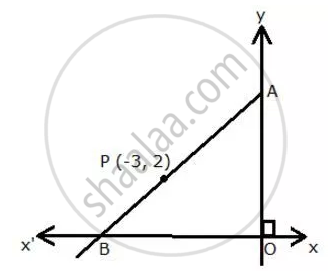Exercise 13 (B) | Q 6 | Page 182

In the given figure, P (4, 2) is mid-point of line segment AB. Find the co-ordinates of A and B.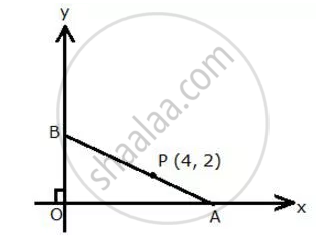Exercise 13 (B) | Q 7 | Page 182

(-5, 2), (3, -6) and (7, 4) are the vertices of a triangle. Find the length of its median through the vertex (3, -6)

Exercise 13 (B) | Q 8 | Page 182

Given a line ABCD in which AB = BC = CD, B= (0, 3) and C = (1, 8)
Find the co-ordinates of A and D.

Exercise 13 (B) | Q 9 | Page 182

One end of the diameter of a circle is (-2, 5). Find the co-ordinates of the other end of it, of the centre of the circle is (2, -1)

Exercise 13 (B) | Q 10 | Page 182

A (2, 5), B (1, 0), C (-4, 3) and D (-3, 8) are the vertices of quadrilateral ABCD. Find the coordinates of the mid-points of AC and BD. Give a special name to the quadrilateral.

Exercise 13 (B) | Q 11 | Page 182

P (4, 2) and Q (-1, 5) are the vertices of parallelogram PQRS and (-3, 2) are the co-ordinates of the point of intersection of its diagonals. Find co-ordinates of R and S.

Exercise 13 (B) | Q 12 | Page 182

A (-1, 0), B (1, 3) and D (3, 5) are the vertices of a parallelogram ABCD. Find the co-ordinates of vertex C.

Exercise 13 (B) | Q 13 | Page 182

The points (2, -1), (-1, 4) and (-2, 2) are mid points of the sides of a triangle. Find its vertices

Exercise 13 (B) | Q 14 | Page 182

Points A (-5, x), B (y, 7) and C (1, -3) are collinear (i.e. lie on the same straight line) such that AB = BC. Calculate the values of x and y.

Exercise 13 (B) | Q 15 | Page 182

Points P (a, −4), Q (−2, b) and R (0, 2) are collinear. If Q lies between P and R, such that PR = 2QR, calculate the values of a and b.

Exercise 13 (B) | Q 16 | Page 182

Calculate the co-ordinates of the centroid of the triangle ABC, if A = (7, -2), B = (0, 1) and C =(-1, 4).

Exercise 13 (B) | Q 17 | Page 182

The co-ordinates of the centroid of a triangle PQR are (2, -5). If Q = (-6, 5) and R = (11, 8); calculate the co-ordinates of vertex P.

Exercise 13 (B) | Q 18 | Page 182

A (5, x), B (-4, 3) and C (y, -2) are the vertices of the triangle ABC whose centroid is the origin. Calculate the values of x and y.

Exercise 13 (C) [Pages 182 - 183]

### Selina solutions for Concise Maths Class 10 ICSE Chapter 13 Section and Mid-Point Formula Exercise 13 (C) [Pages 182 - 183]

Exercise 13 (C) | Q 1 | Page 182

Given a triangle ABC in which A = (4, -4), B = (0, 5) and C = (5, 10). A point P lies on BC such that BP : PC = 3 : 2, Find the length of line segment AP.

Exercise 13 (C) | Q 2 | Page 182

A(20, 0) and B(10, -20) are two fixed points Find the co-ordinates of the point P in AB such that : 3PB = AB, Also, find the co-ordinates of some other point Q in AB such that AB = 6 AQ.

Exercise 13 (C) | Q 3 | Page 183

A(-8, 0), B(0, 16) and C(0, 0) are the verticals of a triangle ABC. Point P lies on AB and Q lies on AC such that AP : PB = 3 : 5 and AQ : QC = 3 : 5

Show that : PQ =3/8 BC

Exercise 13 (C) | Q 4 | Page 183

Find the co-ordinates of points of trisection of the line segment joining the point (6, -9) and the origin.

Exercise 13 (C) | Q 5 | Page 183

A line segment joining A(-1,5/3) and B (a, 5) is divided in the ratio 1 : 3 at P, the point where the line segment AB intersects the y-axis.

(i) calculate the value of ‘a’
(ii) Calculate the co-ordinates of ‘P’.

Exercise 13 (C) | Q 6 | Page 183

In what ratio is the line joining A(0, 3) and B (4, -1) divided by the x-axis? Write the co-ordinates of the point where AB intersects the x-axis

Exercise 13 (C) | Q 7 | Page 183

The mid-point of the segment AB, as shown in diagram, is C(4, -3). Write down the co-ordinates of A and B.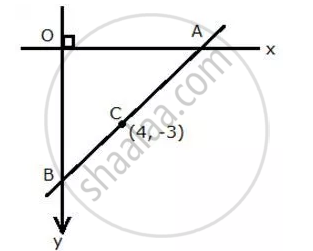Exercise 13 (C) | Q 8 | Page 183

AB is a diameter of a circle with centre C = (-2, 5). If A = (3, -7), find
(i) the length of radius AC
(ii) the coordinates of B.

Exercise 13 (C) | Q 9 | Page 183

Find the co-ordinates of the centroid of a triangle ABC whose vertices are: A(-1, 3), B(1, -1) and C(5, 1)

Exercise 13 (C) | Q 10 | Page 183

The mid point of the line segment joining (4a, 2b -3) and (-4, 3b) is (2, -2a). Find the values of a and b.

Exercise 13 (C) | Q 11 | Page 183

The mid point of the line segment joining (2a, 4) and (-2, 2b) is (1, 2a + 1). Find the values of a and b.

Exercise 13 (C) | Q 12 | Page 183

(i) write down the co-ordinates of the point P that divides the line joining A(-4, 1) and B(17, 10) in the ratio 1 : 2.
(ii) Calculate the distance OP, where O is the origin.
(iii) In what ratio does the Y-axis divide the line AB?

Exercise 13 (C) | Q 13 | Page 183

Prove that the points A(-5, 4); B(-1, -2) and C(5, 2) are the vertices of an isosceles right angled triangle. Find the co-ordinates of D so that ABCD is a square.

Exercise 13 (C) | Q 14 | Page 183

M is the mid-point of the line segment joining the points A(-3, 7) and B(9, -1). Find the coordinates of point M. Further, if R(2, 2) divides the line segment joining M and the origin in the ratio p : q, find the ratio p : q

Exercise 13 (C) | Q 15 | Page 183

Calculate the ratio in which the line joining A(-4,2) and B(3,6) is divided by point p(x,3). Also, find x

Exercise 13 (C) | Q 16 | Page 183

Find the ratio in which the line 2x+y=4 divides th line segment joining the point p(2,-2) and Q (3,7).

Exercise 13 (C) | Q 17 | Page 183

If the abscissa of point P is 2. find the ratio in which this point divides the line segment joining the point (-4,3) and (6,3). Also find the co-ordinates of point P.

Exercise 13 (C) | Q 18 | Page 183

The line joining the points (2,1) and (5,-8) is trisected at the point P and Q, point P lies on the line 2x-y+k=0, find the value of k Also, find the co-ordinates of point Q.

Exercise 13 (C) | Q 19 | Page 183

Find the image of the point A(5,3) under reflection in the point P(-1,3).

Exercise 13 (C) | Q 20 | Page 183

M is the mid-point of the line segment joining the points A(0,4) and B(6,0). M also divides the line segment OP in the ratio 1:3 find :

(a) co-ordinates of M

(b) Co-ordinates of P

(C) Length Of BP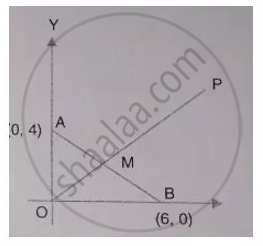Exercise 13 (C) | Q 21 | Page 183

A (-4,2)2, B(0,2) and C (-2,-4) are the vertices of a triangle ABC. A,Q and R are mid-Points of sides BC,CA and AB respectively.Show that the centroid of Δ PQR is the same as the centroid of Δ ABC.

Exercise 13 (C) | Q 22 | Page 183

A(3, 1), B(y, 4) and C(1, x) are vertices of a triangle ABC. P, Q and R are mid - points of sides BC, CA and AB respectively. Show that the centroid of ΔPQR is the same as the centroid ΔABC.

## Chapter 13: Section and Mid-Point Formula

Exercise 13 (A)Exercise 13 (B)Exercise 13 (C)## Selina solutions for Concise Maths Class 10 ICSE chapter 13 - Section and Mid-Point Formula

Selina solutions for Concise Maths Class 10 ICSE chapter 13 (Section and Mid-Point Formula) include all questions with solution and detail explanation. This will clear students doubts about any question and improve application skills while preparing for board exams. The detailed, step-by-step solutions will help you understand the concepts better and clear your confusions, if any. Shaalaa.com has the CISCE Concise Maths Class 10 ICSE solutions in a manner that help students grasp basic concepts better and faster.

Further, we at Shaalaa.com provide such solutions so that students can prepare for written exams. Selina textbook solutions can be a core help for self-study and acts as a perfect self-help guidance for students.

Concepts covered in Concise Maths Class 10 ICSE chapter 13 Section and Mid-Point Formula are Co-ordinates Expressed as (x,y), Distance Formula, Section Formula, The Mid-point of a Line Segment (Mid-point Formula).

Using Selina Class 10 solutions Section and Mid-Point Formula exercise by students are an easy way to prepare for the exams, as they involve solutions arranged chapter-wise also page wise. The questions involved in Selina Solutions are important questions that can be asked in the final exam. Maximum students of CISCE Class 10 prefer Selina Textbook Solutions to score more in exam.

Get the free view of chapter 13 Section and Mid-Point Formula Class 10 extra questions for Concise Maths Class 10 ICSE and can use Shaalaa.com to keep it handy for your exam preparation